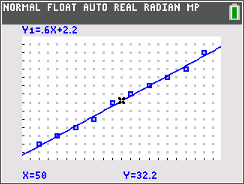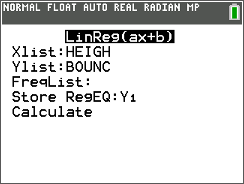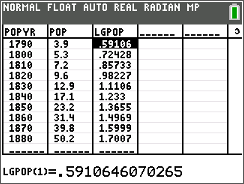# Activities

••• ##### Subject Area

• Math: Statistics: Data Analysis
• Math: Statistics: Describing Bivariate Data

• ##### Author9-12

45 Minutes

• ##### Device
• TI-84 Plus
• TI-84 Plus Silver Edition
•TI-84 Plus C Silver Edition
•TI-84 Plus CE
• ##### Software

TI Connect™ CE

## Transforming Relationships#### Activity Overview

Students assess the strength of a linear relationship using a residual plot and calculate the correlation coefficient and coefficient of determination to assess the data set.

#### Key Steps

•Students are given a program that loads four data sets onto their graphing calculators. They are asked to determine if a linear regression model fits each of the data sets.

•To asses the fit graphically and numerically, students will create a residual plot and look at the r and r2 values.

•Students will transform a data set by calculating the logarithm value of the data, which linearizes the exponential data. They will assess the fit graphically and numerically.